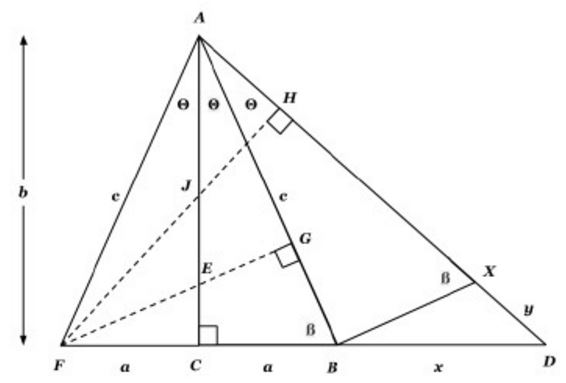# Much from Little

### Introduction

Below is an adaptation of John Molokach's article that he communicated on Friday, 23 December 2016.In the diagram above, let $\Delta ABC\,$ be a right triangle with right angles C and legs $a\,$ and $b.\,$ Label the opposite angles from legs $a\,$ and $b,\,$ $\theta\,$ and $\beta,\,$ respectively. Then construct $\Delta BAX\,$ such that $\angle ABX\,$ is right with $\angle BAX=\theta.\,$ Also extend $AX\,$ and $BC\,$ to their intersection $D\,$ to form $\Delta CAD.\,$ Finally, reflect $\Delta ABC \,$ over $AC\,$ to form $\Delta ACF.$

We shall use this diagram to prove:### $\sin 2\theta=2\sin\theta\cos\theta$The area of $\Delta FAB$ is $[\Delta FAB]=ab=\displaystyle\frac{AB\cdot FG}{2}.\,$ Therefore, $FG=\displaystyle\frac{2ab}{c},\,$ i.e.,

$\sin2\theta=\displaystyle\frac{2ab}{c^2}=2\sin\theta\cos\theta.$

### $\cos 2\theta=2\cos^2\theta-1$From the Angle Bisector Theorem, $\displaystyle\frac{AF}{AG}=\frac{EF}{EG}\,$ so that $AF=\displaystyle\frac{AG\cdot EF}{EG}\,$ and $EF+EG=FG.\,$ Since $\displaystyle\frac{EF}{AB}=\frac{CF}{AC}\,$ also $EF=\displaystyle\frac{ac}{b}.\,$ It follows that $EG=\displaystyle\frac{2ab}{c}-\frac{ac}{b}=\frac{2ab^2-ac^2}{bc}.\,$ Now, since $\displaystyle\frac{EG}{a}=\frac{AG}{b},\,$ it follows that $AG=\displaystyle\frac{2b^2-c^2}{c}.\,$ Therefore, $\cos 2\theta=\displaystyle\frac{AG}{c}=\frac{2b^2-c^2}{c^2}=2\cos^2\theta -1.$

### $a^2+b^2=c^2$Note that, since triangles $ACB\,$ and $ABX\,$ are similar, $\displaystyle\frac{AB}{BX}=\frac{b}{a}.\,$ Also, since triangles $ABD\,$ and $BXD\,$ are similar, $\displaystyle\frac{x}{y}=\frac{AB}{BX},\,$ and so $\displaystyle\frac{x}{y}=\frac{b}{a}=\tan\beta.$

Now, again from the similarity of triangles $ABD\,$ and $BXD,\,$ we have $\displaystyle\frac{AD}{BD}=\frac{x}{y}=\frac{b}{a}.\,$ Therefore, $x=\displaystyle\frac{b}{a}\left(\frac{c^2}{b}+y\right)=\frac{b}{a}\left(\frac{c^2}{b}+\frac{ax}{b}\right),\,$ implying $x=\displaystyle\frac{ac^2}{b^2-a^2}.$

But also, since $\sin 2\theta=\displaystyle\frac{2ab}{c^2}=\frac{a+x}{c^2/b+y}=\frac{a+x}{c^2/b+ax/b},\,$ we have $x=\displaystyle\frac{ac^2}{2b^2-c^2}.\,$ Equating the two expressions for $x\,$ yields $2b^2-c^2=b^2-a^2,\,$ i.e., $a^2+b^2=c^2.$

### $\displaystyle\tan 2\theta=\frac{2\tan\theta}{1-\tan^2\theta}$Dividing both sides of $a^2+b^2=c^2\,$ by $a^2\,$ results in $1+\tan^2\theta=\sec^2\theta.\,$ We can use this fact, along with the expressions above for $\sin 2\theta\,$ and $\cos 2\theta\,$ to write an expression for $\tan 2\theta:$

$\displaystyle\tan 2\theta=\frac{\sin 2\theta}{\cos 2\theta}=\frac{2\sin\theta\cos\theta}{2\cos^2\theta-1}.$

Now dividing the numerator and the denumerator of this last expression by $\cos^2\theta,\,$ we can write

$\displaystyle\tan 2\theta=\frac{2\tan\theta}{2-\sec^2\theta}=\frac{2\tan\theta}{1-\tan^2\theta}.$

### $\sin 3\theta=\sin\theta (3-4\sin^2\theta)$Our goal is to find an expression for $\displaystyle\frac{FH}{AF}.\,$ To do so we find the area of $\Delta FAD\,$ in two ways. On one hand, $[\Delta FAD]=\displaystyle\frac{DF\cdot AC}{2}.\,$ On the other hand, $[\Delta FAD]=\displaystyle\frac{FH\cdot AD}{2}.\,$ This allows us to write

$\displaystyle b(2a+x)=FH\left(\frac{c^2}{b}+\frac{ax}{b}\right).$

Solving for $FH\,$ an using $x=\displaystyle\frac{ac^2}{2b^2-c^2},\,$ we have $FH=\displaystyle\frac{3ab^2-a^3}{c^2}.\,$ Therefore,

$\displaystyle\frac{FH}{AF}=\sin 3\theta=\frac{a}{c}\left(\frac{4b^2-c^2}{c^2}\right)=\sin\theta (4\cos^2\theta -1).$

Now we can divide both sides of $a^2+b^2=c^2\,$ by $c^2\,$ to obtain $\sin^2\theta+\cos^2\theta=1\,$ and use this result to write

$\sin^3\theta=\sin\theta\left(4-\sin^2\theta -1\right)=\sin\theta (3-4\sin^2\theta ).$

### $\cos 3\theta=\cos\theta (4\cos^2\theta-3)$Our goal is to find an expression for $\displaystyle\frac{AH}{c}.\,$ To do so we shall use an equivalent expression: $\displaystyle\frac{AD}{c}-\frac{DH}{c}.\,$ Applying the Angle Bisector Theorem to $\Delta ACD,\,$ we have $\displaystyle\frac{AD}{b}=\frac{x}{a},\,$ so that $\displaystyle\frac{AD}{c}=\frac{bx}{ac}.\,$

Also, from $\Delta FHD\,$ and the double argument formula, derived earlier,

$\displaystyle\cos\angle FHD=\sin 2\theta=\frac{DH}{2a+x}=\frac{2ab}{c^2},$

so that

$\displaystyle\frac{DH}{c}=\frac{2ab(2a+x)}{c^3}.$

This gives us an expression for $\cos 3\theta:$

\displaystyle\begin{align} \cos 3\theta &= \frac{AD}{c}-\frac{DH}{c}=\frac{bx}{ac}-\frac{2ab(2a+x)}{c}\\ &=\frac{b}{c}\left(\frac{x}{a}-\frac{2a(2a+x)}{c}\right)=\frac{b}{c}\left(x\left(\frac{1}{a}-\frac{2a}{c^2}\right)-\frac{4a^2}{c^2}\right)\\ &=\frac{b}{c}\left(x\left(\frac{c^2-2a^2}{ac^2}\right)-\frac{4a^2}{c^2}\right). \end{align}

Now, using $x=\displaystyle\frac{ac^2}{c^2-2ac^2}\,$ from the previous proof, we have

$\displaystyle\cos 3\theta=\frac{b}{c}\left(1-\frac{4a^2}{c^2}\right)=\cos\theta\left(1-4\sin^2\theta\right),$

and once again using $\cos^2\theta+\sin^2\theta=1,\,$ we arrive at our goal:

$\cos 3\theta=\cos\theta\left(4\cos^2\theta-3\right).$

### $\displaystyle\tan 3\theta=\tan\theta\left(\frac{3-\tan^2\theta}{1-3\tan^2\theta}\right)$Now we want to find an expression for $\displaystyle\frac{FH}{AH}\,$ in terms of $a\,$ and $b.\,$ From the previous proof, we can write

$\displaystyle AH=b\left(1-\frac{4a^2}{c^2}\right)=\frac{b}{c^2}(c^2-4a^2).$

With $a^2+b^2=c^2\,$ this gives

$\displaystyle AH==\frac{b}{c^2}(b^2-3a^2).$

From the previous section, $\displaystyle FH=\frac{a}{c^2}(3b^2-a^2)\,$ such that

$\displaystyle \tan 3\theta=\frac{FH}{AH}=\frac{a}{b}\left(\frac{3b^2-a^2}{b^2-3a^2}\right)$

and dividing the numerator and the denumerator by $b^2\,$ we get

$\displaystyle \tan 3\theta=\frac{FH}{AH}=\tan\theta\left(\frac{3-\tan^2\theta}{1-3\tan^2\theta}\right).$

### Trigonometry